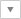...
홈SupportFAQ

FAQ

# GENERAL | How much is N.m when converted to kgf.cm?

Hit 1,620

## 본문

Here is the conversion rate for 1N and 1kgf.
1kgf = 9.8N  <->  1N = 1/9.8 = 0.10197 kgf.
1N.m is 0.102kgf.m  <->  10.2kgf.cm

The AX-12A’s stall torque is 1.5N.m, so
1.5 / 0.098 = about 15.3kgf.cm.

So, when designing using an AX-12A, the load should not exceed 1/5 of the stall torque.
AX-12A’s stall torque = 1.5N.m, so the max. load should be about 0.3N.m (about 3.2kgf.cm).